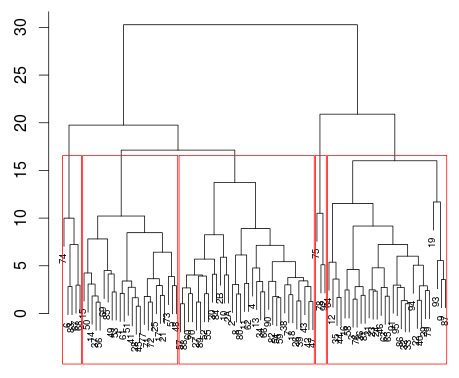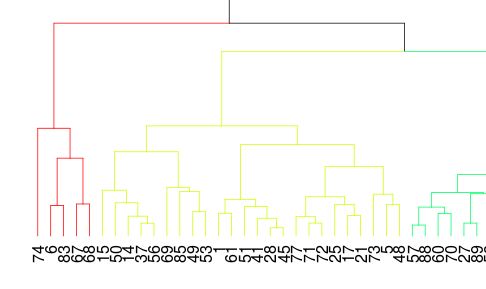Want to share your content on R-bloggers? click here if you have a blog, or here if you don't.

For the data scienec course of tomorrow, I just wanted to post some functions to illustrate cluster analysis. Consider the dataset of the French 2012 elections

```> elections2012=read.table(
> voix=which(substr(names(
+ elections2012),1,11)=="X..Voix.Exp")
> elections2012=elections2012[1:96,]
> X=as.matrix(elections2012[,voix])
> rownames(X)=elections2012[,1]```

The hierarchical cluster analysis is obtained using

```> cah=hclust(dist(X))
> plot(cah,cex=.6)```

To get five groups, we have to prune the tree

```> rect.hclust(cah,k=5)
> groups.5 <- cutree(cah,5)```We have to zoom-in to visualize the French regions,It is also possible to use

```> library(dendroextras)
> plot(colour_clusters(cah,k=5))```And again, if we zoom-in, we getThe interpretation of the clusters can be obtained using

```> aggregate(X,list(groups.5),mean)
Group.1     JOLY   LE PEN  SARKOZY
1       1 2.185000 18.00042 28.74042
2       2 1.943824 23.22324 25.78029
3       3 2.240667 15.34267 23.45933
4       4 2.620000 21.90600 34.32200
5       5 3.140000  9.05000 33.80000```

It is also possible to visualize those clusters on a map, using

```> library(RColorBrewer)
> CL=brewer.pal(8,"Set3")
> carte_classe <- function(groupes){
+ library(stringr)
+ elections2012\$dep <- elections2012[,2]
+ elections2012\$dep <- tolower(elections2012\$dep)
+ elections2012\$dep <- str_replace_all(elections2012\$dep, pattern = " |-|'|/", replacement = "")
+ library(maps)
+ france<-map(database="france")
+ france\$dep <- france\$names
+ france\$dep <- tolower(france\$dep)
+ france\$dep <- str_replace_all(france\$dep, pattern = " |-|'|/", replacement = "")
+ corresp_noms <- elections2012[, c(1,2, ncol(elections2012))]
+ corresp_noms\$dep[which(corresp_noms\$dep %in% "corsesud")] <- "corsedusud"
+ col2001<-groupes+1
+ names(col2001) <- corresp_noms\$dep[match(names(col2001), corresp_noms[,1])]
+ color <- col2001[match(france\$dep, names(col2001))]
+ map(database="france", fill=TRUE, col=CL[color])
+ }
> carte_classe(cutree(cah,5))```or, if we simply want 4 clusters

`> carte_classe(cutree(cah,4))`